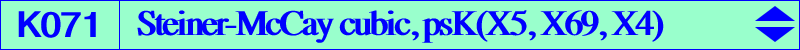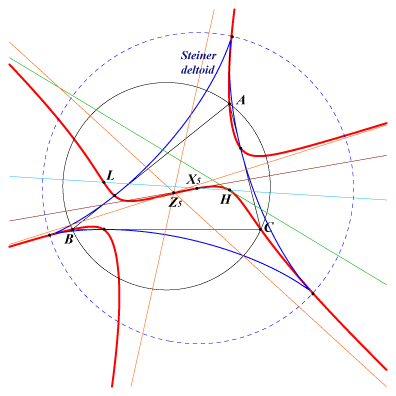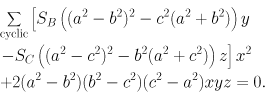X(4), X(5), X(20), X(76), X(5562) cusps of the Steiner deltoid of ABC its contacts with the altitudes of ABC and with the sidelines of ABC (traces of X(69) on the sidelines of ABC) infinite points of the McCay cubic points of pK(X6, X5562) on the circumcircleWe meet this cubic in the FG paper "The Lemoine cubic and its generalizations" §6 under the name Kconc (see the Downloads page). This cubic is a K60+ (i.e. a stelloid) with three real asymptotes parallel to those of the McCay cubic and concurring at Z5 = X(5891), the reflection of X(51) about X(5). K071 is actually the only circum-stelloid passing through the cusps of the Steiner deltoid. We meet its complement in Table 11 : CPCC points related curves. K071 is also a member of the class CL063. See a generalization and the related cubics K648, K649, K650, K651, K652. The isogonal transform of K071 is psK(X25 x X97, X97, X3) = spK(X5562, X1216), a CircumNormal cubic as in Table 25. *** K071 is spK(X3, X1216) as in CL055. The union of the line at infinity with the rectangular circum-hyperbola passing through X(20) and K071 generate a pencil of circum-stelloids spK(X3, Q on the line X550-X1216) which contains K080, X268, K827, K928. All these stelloids have their asymptotes parallel to those of the McCay cubic K003 and concurring on the line X(3)X(64). The remaining common points of these stelloids are X(4), X(20), X(15318). *** K071 is mentioned in Table 64, as the locus of P such that the Lemoine cubic K(P) is a K+. See K041 = K(X20), K054 = K(X5), K056 = K(X76). When P lies on K004, the cubic K(P) is a psK, and then, K041 is a psK+. More generally, K004 and K071 meet at nine points namely A, B, C, H (twice), X(20) and three other points on the circle with center X(5), radius 3R/2. These points give three other psK+. K004 (a pK) and K071 (a stelloid) generate a pencil of cubics which contains K617 (a nodal cubic) and K809 (a circular cubic). Every cubic of the pencil meets (L∞) and (O) at the same points as two isogonal pKs with pivots on the Euler line and the line {20, 2979, 6000, 9833, 12058, 12111, etc} respectively. Recall that K004 and K071 are both K+ and the pencil contains a third K+ passing through X(382) with asymptotes concurring at X(381). It must also contain a third psK but it is complicated with little interest.